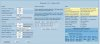# Калкулатори за RC модели

#### st5rov

##### Редовен Потребител
Не знам дали вече има подобна тема. Моля за извинение, ако е така!Ако някой е открил такива програмки нека ги сподели, както направи Tassadar, за избор на витло/http://www.ecalc.ch/motorcalc.php?ecalc&lang=en/.
Ето и една от мен, за изчисление на център тежест:Освен това може да се изчисли и сривната скорост:Последна промяна:

##### Редовен Потребител
Ето тава е много полезна програмка при построяване на модел:

http://flbeagle.rchomepage.com/software/webocalc_1.5.2/html/webocalc_metric.htmlВ процеса на построяване може да добием представа как ще се държи модела при определен размер и тегло, дава насоки за избор на мотор, витло и батерия. На практика преди облитането имате сравнително точна идея как ще лети модела.

#### st5rov

##### Редовен Потребител
:Добавих малко картинки в предния пост!!!Последна промяна:
•vesibo и JetMan

#### geo111

##### Потребител
Е те това е. Знам си че мога да разчитам на вас

•#### st5rov

##### Редовен Потребител
•stalker

#### st5rov

##### Редовен Потребител
•#### st5rov

##### Редовен Потребител
Едно полезен калкулатор с обяснения и формули!

A i r c r a f t S u p e r C a l c u l a t o r 7.5.1

MAC And Neutral Point

use the same units of measure for all entries!The Equations

Wing Sweep, C =
(S * ((2 * B) + A)) / (3 * (A + B))
MAC (length) =
A - (2 * (A - B) * (0.5 * A + B) / (3 * (A + B)))
MAC location, d =
Y * ((A - MAC) / (A - B))
wing area, WA =
2 * Y * ((A + B) / 2)
tail area, TA =
2 * YY * ((AA + BB) / 2)
wing aspect ratio, ARw =
(2 * Y) / ((A + B) / 2)
tail aspect ratio, ARt =
(2 * YY) / ((AA + BB) / 2)
Tail Arm =
(D - wing AC) + tail AC
tail volume, Vbar =
(TA / WA ) * (Tarm / MAC)
NP (%MAC) =
0.25 + (.7 * Vbar * (As / Aw) * ( 1 - de / da));
ideal CG (%MAC) =
NP - Desired Static Margin
actual CG (%MAC) =
((Total Moment / Total Wt) - (Datum to LE + C)) / MAC
actual static margin % =
(NP - actual CG) / MAC * 100
Center of Gravity (CG) is the point where the weight of the aircraft is balanced.

Neutral Point (NP) is the point where the aerodynamic forces of the wing and tail are balanced.

25% - 35% MAC is the typical range for the CG of a conventional aircraft .

Placing CG 5% to 15% of MAC in front of NP creates a longitudinal (pitch) stability called Static Margin. A lower margin (tail heavy) produces less stability and greater elevator authority, while a higher margin (nose heavy) creates more stability and less elevator authority. A static margin that's too high may result in elevator stall at take off and landing.

Vbar is a unitless volume coefficient, based on the ratio of the wing surface area to the horizontal tail group (stabilizer and elevator) surface area and the distance between the wing's AC and the tail's AC. It quantifies the tail's aerodynamic effectiveness and is used to calculate NP. Typical values range from 0.35 (smaller or closer to the wing, thus less effective) to 0.6 (larger or farther from the wing, thus more effective).

•arsovhome и Xape